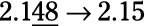##### Basic Math & Pre-Algebra Workbook For Dummies with Online Practice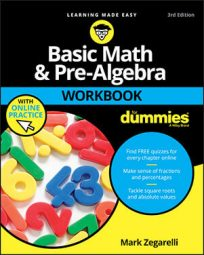Dividing decimals is similar to dividing whole numbers, except you have to handle the decimal point before you start dividing. Here’s how to divide decimals step by step:
1. Move the decimal point in the divisor and dividend.

Turn the divisor (the number you’re dividing by) into a whole number by moving the decimal point all the way to the right. At the same time, move the decimal point in the dividend (the number you’re dividing) the same number of places to the right.

2. Place a decimal point in the quotient (the answer) directly above where the decimal point now appears in the dividend.

3. Divide as usual, being careful to line up the quotient properly so that the decimal point falls into place.

Line up each digit in the quotient just over the last digit in the dividend used in that cycle.

As with whole-number division, sometimes decimal division doesn’t work out evenly at the end. With decimals, however, you never write a remainder. Instead, attach enough trailing zeros to round the quotient to a certain number of decimal places. The digit to the right of the digit you’re rounding to tells you whether to round up or down, so you always have to figure out the division to one extra place.

See the following chart:
To Round a Decimal to Fill Out the Dividend with Trailing Zeros to
A whole number One decimal place
One decimal place Two decimal places
Two decimal places Three decimal places

## Sample questions

1. Divide the following: 9.152 / 0.8 = ?

11.44. To start out, write the problem as usual: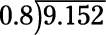Turn 0.8 into the whole number 8 by moving the decimal point one place to the right. At the same time, move the decimal point in 9.1526 one place to the right. Put your decimal point in the quotient directly above where it falls in 91.25: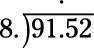Now you’re ready to divide. Just be careful to line up the quotient properly so that the decimal point falls into place.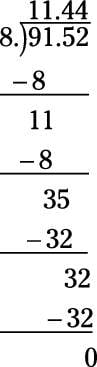2. Divide the following: 21.9 / 0.015 = ?

1,460. Set up the problem as usual: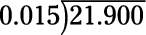Notice that two trailing zeros are attached to the dividend because you need to move the decimal points in each number three places to the right. Again, place the decimal point in the quotient directly above where it now appears in the dividend, 21900: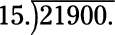Now you’re ready to divide. Line up the quotient carefully so the decimal point falls into place: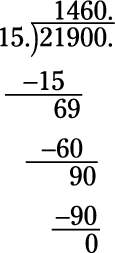Even though the division comes out even after you write the digit 6 in the quotient, you still need to add a placeholding zero so that the decimal point appears in the correct place.

## Practice questions

1. Divide these two decimals: 9.345 / 0.05 = ?

2. Solve the following division: 3.15 / 0.021 = ?

3. Perform the following decimal division, rounding to one decimal place: 6.7 / 10.1.

4. Find the solution, rounding to the nearest hundredth: 9.13 / 4.25.

Following are answers to the practice questions:
1. 9.345 / 0.05 = 186.9. To start out, write the problem as usual: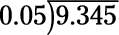Turn the divisor (0.05) into a whole number by moving the decimal point two places to the right. At the same time, move the decimal point in the dividend (9.345) two places to the right. Place the decimal point in the quotient directly above where it now appears in the dividend: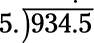Now you’re ready to divide. Be careful to line up the quotient properly so that the decimal point falls into place.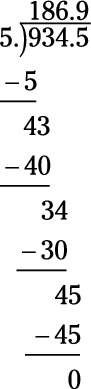2. 3.15 / 0.021 = 150. Write the problem as usual: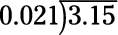You need to move the decimal point in the divisor (0.021) three places to the right, so attach an additional trailing zero to the dividend (3.15) to extend it to three decimal places: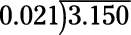Now you can move both decimal points three places to the right. Place the decimal point in the quotient above the decimal point in the dividend: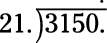Divide, being careful to line up the quotient properly: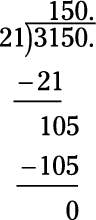Remember to insert a placeholding zero in the quotient so that the decimal point ends up in the correct place.

3. 6.7 / 10.1 = 0.7. To start out, write the problem as usual: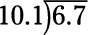Turn the divisor (10.1) into a whole number by moving the decimal point one place to the right. At the same time, move the decimal point in the dividend (6.7) one place to the right: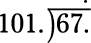The problem asks you to round the quotient to one decimal place, so fill out the dividend with trailing zeros to two decimal places: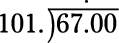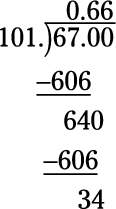Round the quotient to one decimal place: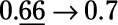4. 9.13 / 4.25 = 2.15. First, write the problem as usual: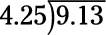Turn the divisor (4.25) into a whole number by moving the decimal point two places to the right. At the same time, move the decimal point in the dividend (9.13) two places to the right: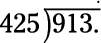The problem asks you to round the quotient to the nearest hundredth, so fill out the dividend with trailing zeros to three decimal places: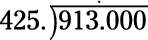Now divide, carefully lining up the quotient: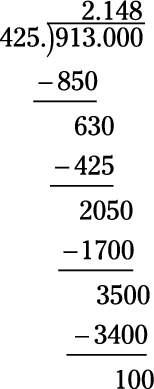Round the quotient to the nearest hundredth: